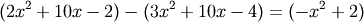# numpy.polysub¶

numpy.polysub(a1, a2)

Difference (subtraction) of two polynomials.

Given two polynomials a1 and a2, returns a1 - a2. a1 and a2 can be either array_like sequences of the polynomials’ coefficients (including coefficients equal to zero), or poly1d objects.

Parameters: a1, a2 : array_like or poly1d Minuend and subtrahend polynomials, respectively. out : ndarray or poly1d Array or poly1d object of the difference polynomial’s coefficients.

Examples```>>> np.polysub([2, 10, -2], [3, 10, -4])
array([-1,  0,  2])
```

numpy.polymul

#### Next topic

numpy.RankWarning